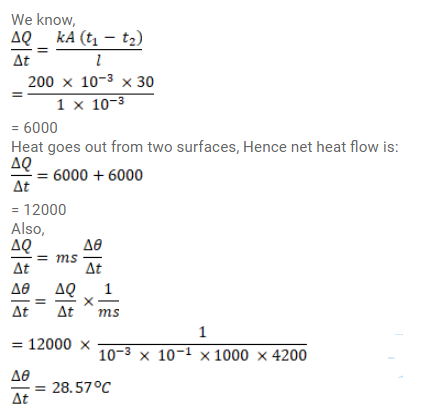# Water at 50°C is filled in a closed cylindrical vessel of height 10cm

Question:

Water at $50^{\circ} \mathrm{C}$ is filled in a closed cylindrical vessel of height $10 \mathrm{~cm}$ and cross - sectional area $10 \mathrm{~cm}^{2}$. The walls of the vessel are adiabatic but the flat parts are made of $1 \mathrm{~mm}$ thick Aluminium $\left(\mathrm{K}=200 \mathrm{~J} / \mathrm{m}-\mathrm{s}^{\circ} \mathrm{C}\right)$. Assume that the outside temperature is $20^{\circ} \mathrm{C}$. The density of water is $1000 \mathrm{~kg} / \mathrm{m}^{3}$, and the specific heat capacity of water $=4200 \mathrm{~J} / \mathrm{kg}^{\circ} \mathrm{C}$. Estimate the time taken for the temperature to fall by $1.0^{\circ} \mathrm{C}$. Make any simplifying assumptions you need but specify them.

Solution: